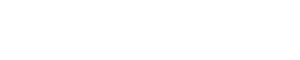# Basic Thermodynamics

#### Course Topics

• INTRODUCTION
• Thermodynamics Definitions
• Thermodynamics and Energy
• Application Areas of Thermodynamics
• State and Equilibrium
• Properties of State
• Forms of Energy
• Systems
• First Law of Thermodynamics and its Application
• Energy Balance and Mechanism of Energy Transfer
• Steady Flow Energy Equation & Non Flow Energy Equation
• Energy Conversion Efficiencies
• Properties of Pure Substances and Gases
• Phase Change Processes of Pure Substances
• Property Diagrams for Phase - Change Processes
• Pressure-Temperature Diagram
• Pressure - Volume Diagram
• Temperature - Volume Diagram
• Temperature - Entropy Diagram
• Enthalopy-entropy diagram or Mollier diagram.
• Use of Steam Tables and Steam Charts
• Compressibility Chart
• Equation of State
• Second Law of Thermodynamics
• Introduction to the second law of Thermodynamics
• Clausius Statement of the Second Law
• Perpetual Motion Machines, Reversible & Irreversible Processes
• The Carnot Cycle & Principles, Thermodynamic Temperature Scale
• Ideal Cycles
• Air Standard Otto Cycle
• AIR STANDARD DIESEL CYCLE
• AIR STANDARD DUAL CYCLE
• Reciprocating engine overview
• Main components of reciprocating IC engines
• Four stroke engine
• Two stroke engine
• TESTING AND PERFORMANCE
• Vapour Power Cycles
• Simple Rankine Cycle
• Practical Rankine Cycle
• Reheat Cycle
• Regenerative Cycle
• Binary Vapor Cycle
• Gas Mixtures
• Dalton 's Law of partial pressure
• Amagat's Law:
• Therdynamic Properties of Mixtures

#### INTRODUCTION

The most of general sense of thermodynamics is the study of energy and its relationship to the properties of matter. All activities in nature involve some interaction between energy and matter. Thermodynamics is a science that governs the following:

• Energy and its transformation
• Feasibility of a process involving transformation of energy
• Feasibility of a process involving transfer of energy
• Equilibrium processes

More specifically, thermodynamics deals with energy conversion, energy exchange and the direction of exchange.